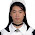-->

## 2008/01/08

### 計概考題

`int a = 0;int b = 1;if ((a=1) || (b=2)) {    printf("Hello, a=%d, b=%d", a, b);}else {    printf("World! a=%d, b=%d", a, b);}`

#### 10 則留言:

1.這是"對"的
if裡面只要是"評算式"就是合法
在a被指定完以後它會評算a的值

短路評算也是C標準規定的

2.題意就跟小學時候的改錯用意一樣囉

3.這不是計概老師出的啦，是外面在應徵Software Engineer的題目

4.這語法應該是成立的吧!?
考你熟析度吧!?

btw, 這是C ? 還是 C like ?

5.這是C
把(a=1) || (b=2)改成(1=a) || (2=b)
這樣在compile time就會出錯
必須改成(1==a) || (2==b)才能通過

6.「球關」應該是「loveflames」吧
就算這是面試考題也跟計概有關，不過這到是蠻有意思的。

這你所說的 1=a 在編譯上一定是錯誤，不過應該不會有人這樣做來降低可讀性吧？

7.1==a的寫法其實很常用
這是防止typo的寫法
a == 1錯打成a = 1在compile time不會出錯
但是1 == a錯打成1 = a就可以馬上發現

8.好聰明的技倆……記起來！

9.路人來亂入一下

會不會是也考Short-circuit evaluation...
XD

順便機會教育一下（眾毆）

10.Short-circuit evaluation